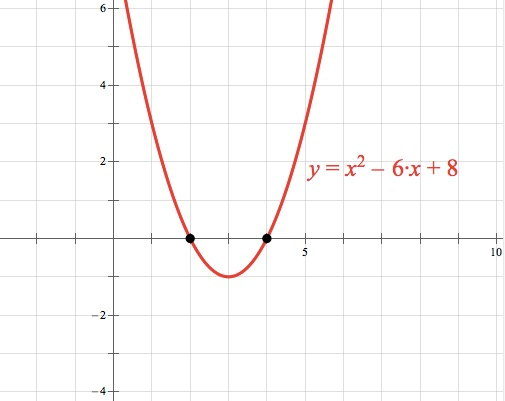Test 2 Section 4 #7

When you get one of these “equivalent forms” questions, especially in a calculator section, you have lots of options. But before we get to those, let’s just make sure we understand what the question is asking.

“Which of the following equivalent forms of the equation…” means that ALL FOUR CHOICES should be equivalent—if you graph them all you’ll get the same parabola!

“…displays the x-intercepts of the parabola as constants or coefficients?” means that you’re looking for the form that has the actual numbers of both the parabola’s x-intercepts in the equation itself.

Now, here’s where your options come in.

First and fastest, if you know your parabola forms well, you know that the factored form,gives you the x-intercepts (they’re at a and b). Recognize that and you’re down to choice D right away.

If you don’t know that (or if you don’t trust your memory of arcane parabola forms), you can always graph the given equation, see its x-intercepts, and then look for those numbers!Looks like the x-intercepts are at 2 and 4, so find the choice that has both a 2 and a 4 in it! Only choice D does, so that’s the answer.